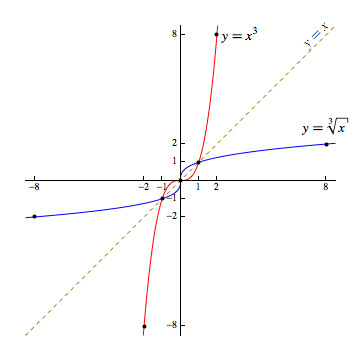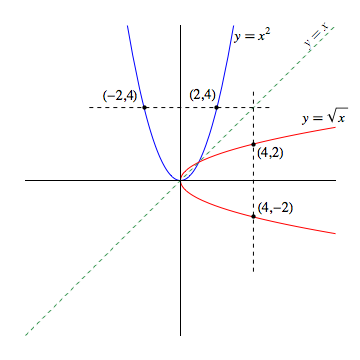## Inverse Functions

Two functions are inverses of one another if they "undo each other" in the following sense: if the output of one is used as input to the other, they leave the original input unchanged.

To be precise, two functions $f$ and $g$ are inverses of each other if and only if $f(g(x)) = x$ for every value of $x$ in the domain of $g$ and $g(f(x)) = x$ for every value of $x$ in the domain of $f$.

Example

Given $f(x) = x^3$ and $g(x) = \sqrt{x}$, we know $f$ and $g$ are inverses since

$$\begin{array}{rcccccl} f(g(x)) &=& f(\sqrt{x}) &=& (\sqrt{x})^3 &=& x \quad \textrm{and}\\ g(f(x)) &=& g(x^3) &=& \sqrt{x^3} &=& x \end{array}$$

We also say a function $f$ is invertible if there exists a function $g$ such that $f$ and $g$ are inverses of one another.

### Notation for Inverse Functions

Historically, we let $f^{-1}$ denote the inverse of an invertible function $f$.

A common source of confusion for students stems from how similar this notation looks to exponentiation.

However, if we wish to apply a power of $-1$ to something, that something must be a value. The letter $f$ represents a function, not a value!

On the other hand, $f(x)$ is a value -- it's the output of function $f$ given an input of $x$. So $f(x)$ actually can be raised to the $-1$ power...

Putting these thoughts together into an example, suppose $f(x)=x^3$. Then,

$$f^{-1}(x) = \sqrt{x} \quad \quad \textrm{while,} \quad \quad [f(x)]^{-1} = \frac{1}{x^3}$$

### Graphs of Inverse Functions

We have already established that $f(x)=x^3$ and $g(x)=\sqrt{x}$ are inverses. Let us consider the graphs of these two functions, as shown below:There is a striking symmetry between these two graphs -- one that is shared between any pair of inverse functions we might choose to draw.

In every case, two functions that are inverses of one another will be symmetric about the line $y=x$.

Equivalently, if $(x,y)$ will be a point on the graph of some invertible function $f$ if and only if $(y,x)$ is a point on the graph of $y=f^{-1}(x)$.

Just consider the graphs of $f(x)=x^3$ and $g(x)=\sqrt{x}$ shown above. The points $(-2,-8)$, $(-1,-1)$, $(0,0)$, $(1,1)$, and $(2,8)$ are all points on the graph of the former. While $(-8,-2)$, $(-1,-1)$, $(0,0)$, $(1,1)$, and $(8,2)$ are all on the graph of the latter.

To see why this is so, suppose $(x_0,y_0)$ is a point on the graph of $y=f(x)$ and $f$ is invertible.

Consequently, $f(x_0) = y_0$, and thus, $f^{-1}(f(x_0)) = f^{-1}(y_0)$.

However, since $f^{-1}$ is the inverse of $f$, we also know $f^{-1}(f(x_0))=x_0$.

Hence, $f^{-1}(y_0) = x_0$, which tells us that $(y_0,x_0)$ is a point on the graph of $y=f^{-1}(x)$.

Since every point $(x,y)$ on the graph of an invertible function yields a point $(y,x)$ on the graph of its inverse and vice-versa, and given that the domain of a function is the set of allowable inputs $x$, and the range is the set of obtainable outputs $y$ -- we have as an immediate consequence that the domain of an invertible function is the range of its inverse, and the range of such a function is the domain of its inverse.

### The Horizontal Line Test

Not every function is invertible. Fortunately, we can tell which functions are invertible from those that are not by a quick examination of their graphs.

As an example, suppose you are interested in determining whether or not $f(x)=x^2$ is invertible.

Keeping in mind the aforementioned symmetry between inverse functions about the line $y=x$, suppose we graph both $y=x^2$ (shown in blue below) and its reflection about the line $y=x$ (shown in red below).Notice, the red curve doesn't pass the vertical line test! That is to say, there is a vertical line that crosses the red curve more than once [e.g., the line through $(4,2)$ and $(4,-2)$].

Equivalently, there is at least one $x$ (e.g., $x=4$) that is associated with more than one $y$-value (e.g., both $2$ and $-2$). Consequently, the red curve does not represent a function.

As the inverse to a function must by definition be a function itself, it must be the case that $f(x)=x^2$ is not invertible.

Note, we could have been more efficient by realizing that any intersections along a vertical line in the graph of the reflection across the line $y=x$ must correspond to intersections along a horizontal line in the graph of $y=f(x)$.

Thus, if any horizontal line crosses the graph of $y=f(x)$ more than once, then $f$ will not have an inverse function associated with it, and is consequently not invertible.

Consequently, if a function is invertible it must be the case that for every $x$ value in the domain, there is one and only one $y$ value associated with that $x$ value, and for every $y$ value in the range, there is one and only one $x$ value associated with that $y$ value. As such, we also describe invertible functions as functions that are one-to-one.

### Solving for an Inverse Function

In certain circumstances, given a formula for some invertible function $f$, we can determine a formula for its inverse $f^{-1}$.

To do this, we take advantage of the previously discovered symmetry between the coordinates of points on the graphs of a function and its inverse, as the following suggests:

Suppose we wished to find a formula for the inverse of a function $f$ defined by $\displaystyle{f(x)= \frac{1+x}{1-2x}}$.

First, we note that if $(x,y)$ is a point on the graph of $f^{-1}$, then $(y,x)$ must be a point on the graph of $f$.

Then, it must be the case that

$$\displaystyle{\begin{array}{rcl}x &=& \displaystyle{\frac{1+y}{1-2y}}\end{array}}$$

From here, we can simply solve for $y$, which then gives us a formula for $f^{-1}(x)$:

$$\begin{array}{rcl} x(1-2y) &=& 1+y\\\\ x - 2xy &=& 1+y\\\\ y+2xy &=& 1-x\\\\ y(1+2x) &=& 1-x\\\\ y &=& \displaystyle{\frac{1-x}{1+2x}} \quad \rightarrow \quad f^{-1}(x) = \displaystyle{\frac{1-x}{1+2x}} \end{array}$$